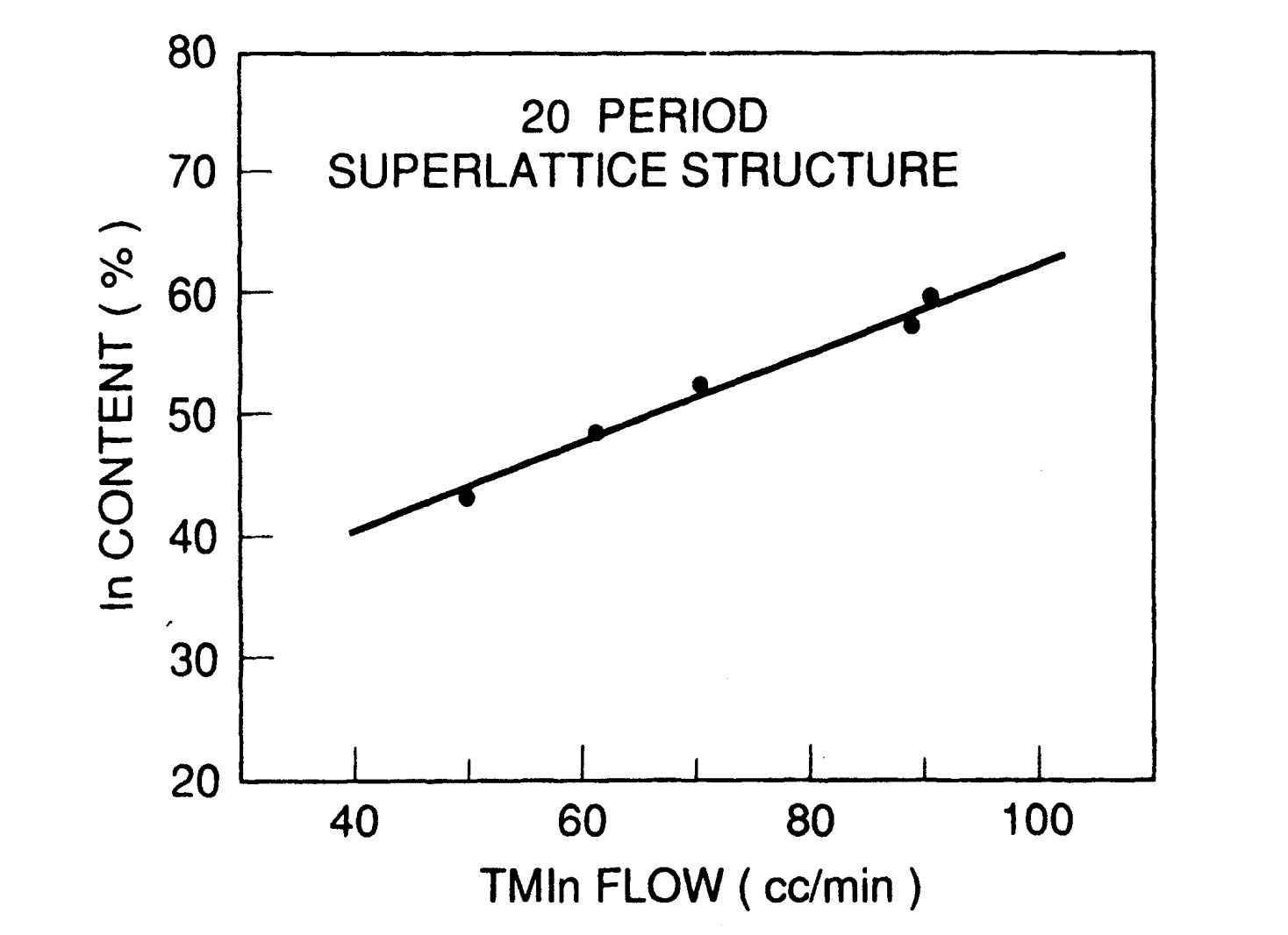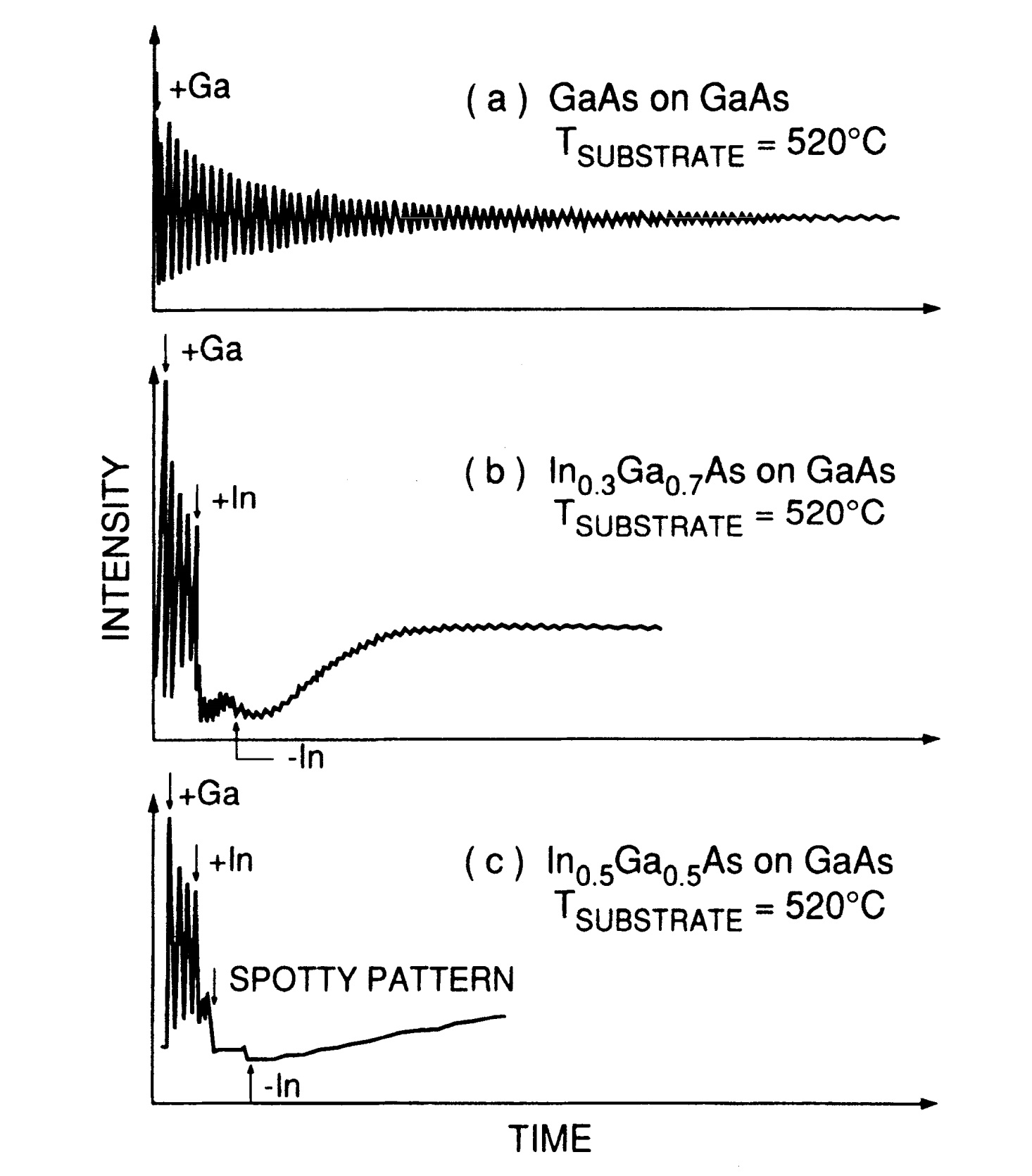# What is Strained-Layer Epitaxy?

This is a continuation from the previous tutorial - material parameters of InGaAsP quaternary alloy grown on InP.

Although the growth of lattice-matched layers is very important for the fabrication of reliable semiconductor lasers, it is possible to make high-quality semiconductor lasers using materials with a small degree of lattice mismatch among them.

This lattice mismatch introduces strain on the epitaxial layers, altering the semiconductor band structure. Typical values of tolerable strain ($$\Delta{a}/a$$, where $$a$$ is the lattice constant of the substrate and $$\Delta{a}$$ is the difference in lattice constants between the substrate and the epitaxial layer) are less than 1.5%.

The larger the amount of strain, the thinner is the layer that can be grown free of dislocations on the substrate. Beyond a certain thickness, known as the critical thickness, very large numbers of dislocations are generated, and the luminescence properties of the material become quite poor.

The two material systems that have been studied extensively for the fabrication of strained-layer lasers are

(i) InGaAsP active region grown over InP

(ii) InGaAs active region grown over GaAs

The former emits in the wavelength range of 1.2-1.6 μm whereas the latter covers the wavelength range of 0.85-1.05 μm. Both types of strained-layer lasers have some advantages discussed later in the context of quantum-well devices.

The InGaAsP semiconductor alloy can also be grown lattice-matched on InP as discussed in the previous tutorials.

Non-lattice-matched layers have been grown mainly by using MOVPE and CBE growth techniques. Layer composition can be changed by varying the flow rate of one of the species.

Changes in the indium concentration in the strained $$\text{In}_{1-x}\text{Ga}_x\text{As}$$ solid as a function of trimethylindium (TMIn) flow are shown in Figure 4-25.Figure 4-25.  Indium concentration in $$\text{In}_{1-x}\text{Ga}_x\text{As}$$ epitaxial layer grown over InP as a function of TMIn flow.

For the layer lattice-matched to InP, the In content is 53%. The data were obtained from superlattice samples grown over the (100)-oriented InP substrate by atmospheric pressure MOVPE, containing 20-50 periods of strained quantum wells separated by 20-nm thick InP barriers.

Strained layers retain their structural integrity as judged by sharp and intense satellite peaks in x-ray diffraction measurements. The linear relationship between TMIn flux and solid-state In composition in $$\text{In}_{1-x}\text{Ga}_x\text{As}$$ suggest TMIn is completely decomposed at the growth temperature ($$625^\circ\text{C}$$) and is proportionally incorporated in the epitaxial $$\text{In}_{1-x}\text{Ga}_x\text{As}$$ layer.

Strained $$\text{In}_{1-x}\text{Ga}_x\text{As}$$ layers have been grown on GaAs substrates using both MBE and MOVPE growth techniques. When the growth takes place by MBE, it is possible to monitor the growth front in situ using RHEED (reflection high-energy electron diffraction) oscillations. Figure 4-26 shows RHEED oscillations for the three cases (a) GaAs on GaAs, (b) $$\text{In}_{0.3}\text{Ga}_{0.7}\text{As}$$ on GaAs, and (c) $$\text{In}_{0.5}\text{Ga}_{0.5}\text{As}$$ on GaAs.Figure 4-26.  RHEED-oscillation data for growth of (a) GaAs on GaAs, (b) $$\text{In}_{0.3}\text{Ga}_{0.7}\text{As}$$ on GaAs, and (c) $$\text{In}_{0.5}\text{Ga}_{0.5}\text{As}$$ on GaAs. "+" and "-" represent the addition or removal of those species, e.g., +Ga means Ga beam is turned on at the time indicated.

In the first case, which corresponds to the lattice-matched growth, RHEED oscillations show a smooth layer-by-layer growth.

However, in the strained case (when the In source is turned on) the growth mode changes rapidly to one that suggests island formation, i.e., RHEED oscillations disappear.

As the strain is increased (higher In mole fraction), the transition to the island-growth mode becomes more abrupt, as seen in Figure 4-26(c).

The next tutorial discuss the characteristics of broad-area lasers